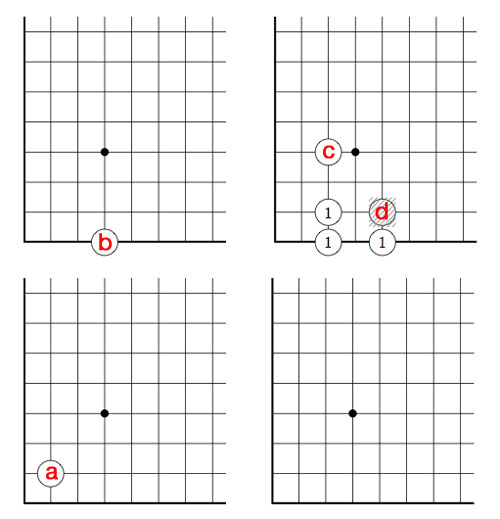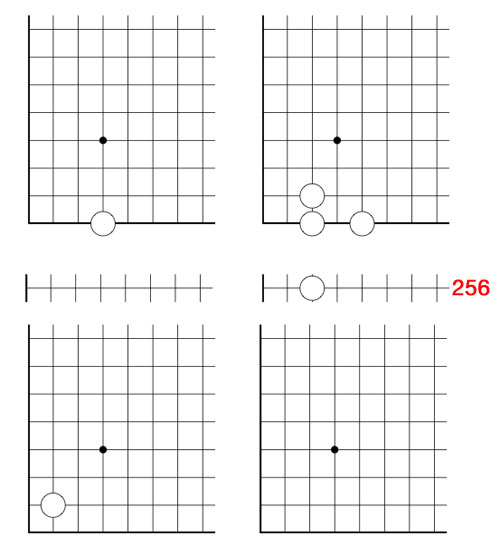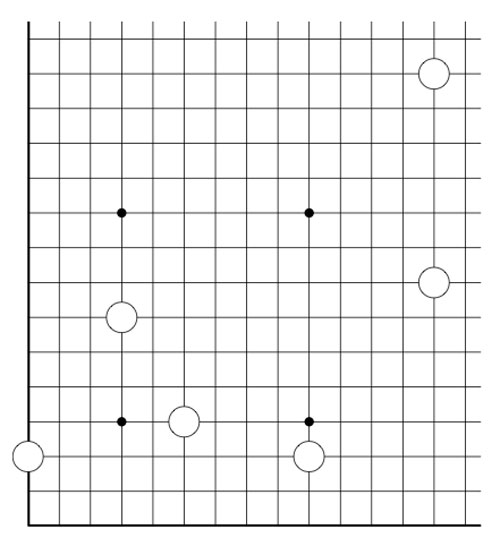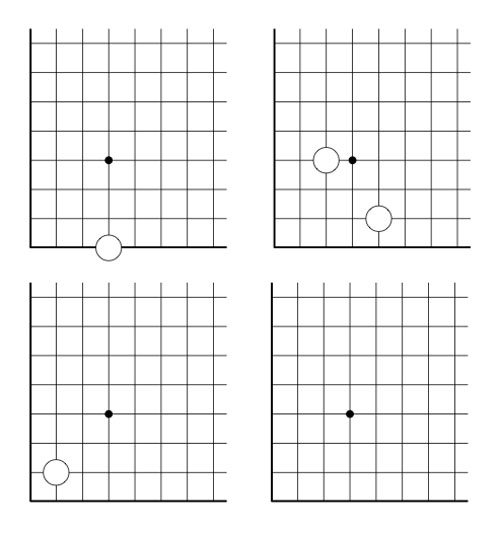# Tag: transfinite numbers

Last time we introduced the game of transfinite Nimbers and asked a winning move for the transfinite game with stones a at position $~(2,2)$, b at $~(4,\omega)$, c at $~(\omega+2,\omega+3)$ and d at position $~(\omega+4,\omega+1)$.Above is the unique winning move : we remove stone d and by the rectangle-rule add three new stones, marked 1. We only need to compute in finite fields to solve this and similar problems.

First note that the largest finite number occuring in a stone-coordinate is 4, so in this case we can perform all calculations in the field $\mathbb{F}_{2^{2^2}}(\omega) = \mathbb{F}_{2^{12}}$ where (as before) we identify $\mathbb{F}_{2^{2^2}} = { 0,1,2,\ldots,15 }$ and we have seen that $\omega^3=2$ (for ease of notation all Nim-additions and Nim-multiplications are denoted this time by + and x instead of $\oplus$ and $\otimes$ as we did last time, so for example $\omega^3 = \omega \otimes \omega \otimes \omega$).

If you’re not nimble with the Nim-tables, you can check all calculations in SAGE where we define this finite field via

sage: R.< x,y,z>=GF(2)[]
sage: S.< t,f,o>=R.quotient((x^2+x+1,y^2+y+x,z^3+x))

and we can now calculate in $\mathbb{F}_{2^{12}}$ using the symbols t for Two, f for Four and o for $\omega$. For example, we have seen that the nim-value of a stone is the nim-multiplication of its coordinates

sage: t*t
t + 1
sage: f*o
f*o
sage: (o+t)*(o+t+1)
o^2 + o + 1
sage: (o+f)*(o+1)
f*o + o^2 + f + o

That is, the nim-value of stone a is 3, stone b is $4 \times \omega$, stone c is $\omega^2+\omega+1$ and finally that of stone d is equal to $\omega^2+5 \times \omega +4$.

By adding them up, the nim-value of the original position is a finite number : 6. Being non-zero we know that the 2nd player has a winning strategy.

Just as in ordinary nim, we compare the value of a stone to the sum of the values of the other stones, to determine the stone we will play. These sums are for the four stones : 5 for a, $4 \times \omega+6$ for b, $\omega^2+\omega+7$ for c and $\omega^2+5 \times \omega+2$ for d. There is only one stone where this sum is smaller than the stone-value, so we know we have to make our move with stone d.

By the Nimbers-rule we need to find a rectangle with top-right hand corner $~(\omega+4,\omega+1)$ and lower-left hand corner $~(u,v)$ such that the values of the three new corners adds up to $\omega^2+5 \times \omega+2$, that is we have to solve

$u \times v + u \times (\omega+1) + (\omega+4)\times v = \omega^2+5 \times \omega+2$

where u and v are ordinals smaller than $\omega+4$ and $\omega+1$. u and v cannot be both finite, for then we wouldn’t obtain a $\omega^2$ term. Similarly (u,v) cannot be of the form $~(u,\omega)$ with u finite because then the left-hand sum would be $\omega^2+4 \times \omega + u$ and likewise it cannot be of the form $~(\omega+i,v)$ with i and v finite as then the coefficient of $\omega$ in the left-hand sum will be i+1 and we cannot take i equal to 4.

The only remaining possibility is that (u,v) is of the form $~(\omega+i,\omega)$ with i finite, in which case the left-hand sum equals $~\omega^2+ 5 \times \omega + i$ whence i=2 and we have found our unique winning move!

But, our opponent can make life difficult by forcing us to compute in larger and larger finite fields. For example, if she would move next by dropping the c stone down to the 256-th row, what would be our next move?(one possible winning move is to remove the stone at $~(\omega+2,\omega)$ and add stones at the three new corners of the rectangle : $~(257,2), (257,\omega)$ and $~(\omega+2,2)$)

Today, we will expand the game of Nimbers to higher dimensions and do some transfinite Nimber hacking.

In our identification between $\mathbb{F}_{16}^*$ and 15-th roots of unity, the number 8 corresponds to $\mu^6$, whence $\sqrt{8}=\mu^3=14$. So, if we add a stone at the diagonal position (14,14) to the Nimbers-position of last timewe get a position of Nim-value 0, that is, winnable for the second player. In fact, this is a universal Nimbers-truth :

Either the 2nd player wins a Nimbers-position, or one can add one stone to the diagonal such that it becomes a 2nd player win.

The proof is elementary : choose a Fermat 2-power such that all stones have coordinates smaller than $2^{2^n}$. If the Nim-value of the position isn’t zero, it corresponds to a unit $\alpha \in \mathbb{F}_{2^{2^n}}^*$. Now,the Frobenius map $x \mapsto x^2$ is an automorphism of any finite field of characteristic two, so the square root $\sqrt{\alpha}$ also belongs to $\mathbb{F}_{2^{2^n}}$, done!

3-dimensional Nimbers is played in the first octant of the integral lattice $\mathbb{Z}^3$ by placing a finite number of balls at places $~(a,b,c) \in \mathbb{N}^3$ with $abc \geq 1$.

Moves are defined by replacing the rectangular-rule of the two-dimensional version by the cuboid-rule : take a cuboid with faces parallel to the coordinate planes whose corner of maximal distance from the origin is one of the balls in the position. Remove that ball and add new balls to the unoccupied corners and remove balls at occupied corners.

Here, we allow the corner-points to have zero as some of its coordinates, but these balls are considered dead in the game. As in the two-dimensional game, this cuboid-rule encompasses several legal moves depending on the number of corners in the cuboid having zero-coordinates.

Again, it follows by induction that the Nim-value of a ball placed at position $~(a,b,c)$ is equal to the Nim-multiplication $a \otimes b \otimes c$ and we can calculate the Nim-value of a 3-dimensional Nimbers-position by computing in a suitable field $\mathbb{F}_{2^{2^n}}$. (The extension to higher dimensions is now obvious)

Does 3-dimensional Nimbers satisfy the ‘universal truth’, that is, can one make any position a 2nd player win by adding at most one stone to the body-diagonal?

The previous argument fails. As $\mathbb{F}_4^*$ is the cyclic group of order three, the 3rd roots of unity in $\overline{\mathbb{F}_2}$ correspond to the numbers 1,2 and 3, so the map $x \mapsto x^3$ cannot be a bijection on any of the finite fields $\mathbb{F}_{2^{2^n}}$.

But then, perhaps, a third root is added by going to a larger such field $\mathbb{F}_{2^{2^N}}$? Well, not quite. Take for example 2, then $\sqrt{2} \notin \mathbb{F}_{2^{2^N}}$. (2 has order 3 in $\mathbb{F}_4$ and so its 3rd root must have order 9, but 9 does not divide any number of the form $2^{2^N}-1$ as the Fermat-powers mod 9 can only be 4 or 7).

In fact one can show that this also holds for any number not in the image of the cubing-map in some $\mathbb{F}_{2^{2^n}}$ as

$\mathbb{N}={ 0,1,2,\ldots } = \cup_N \mathbb{F}_{2^{2^N}}$

with Nim-addition and multiplication is the quadratic closure of $\mathbb{F}_2$ (see for example ONAG).

The situation changes if we allow ourself to play transfinite Nimbers, with the same rules as before but now we allow the stone, balls etc. to be placed at points of which the coordinates are not restricted to $\mathbb{N}_+ = \{ 1,2,3,\ldots \}$ but may vary over $[\beta]_+$ for some ordinal $\beta$ where $[\beta]_+=\{ 1,2,\ldots,\omega,\omega+1,\ldots \}$ is the set of all ordinals smaller than $\beta$.

In transfinite 3-dimensional Nimbers the ‘universal truth’ still holds, provided we play it on a cube of sizes $[\omega^{\omega}]_+$. In particular we have that $\sqrt{2}=\omega$ by the simplicity rule (see ONAG or the Conway’s nim-arithmetic post)

In general, n-dimensional transfinite Nimbers played on an n-gid of sizes $[\omega^{\omega^{\omega}}]_+$ satisfies the universal truth : either a position is a 2nd player win or it becomes one by adding one n-ball to a diagonal position! (this follows immediately because $[\omega^{\omega^{\omega}}]$ with Nim-addition and multiplication is isomorphic to the algebraic closure of $\mathbb{F}_2$).

2-dimensional transfinite Nimbers is still pretty playable. Below a position on a $\omega.2$-board with stones as positions $~(2,2),(4,\omega),(\omega+2,\omega+3)$ and $~(\omega+4,\omega+1)$Give a winning move for the first player!